# seaborn 绘制分类图

### 准备工作

import numpy as np
import pandas as pd
from pandas import Series, DataFrame
import matplotlib.pyplot as plt
import seaborn as sns
%matplotlib inline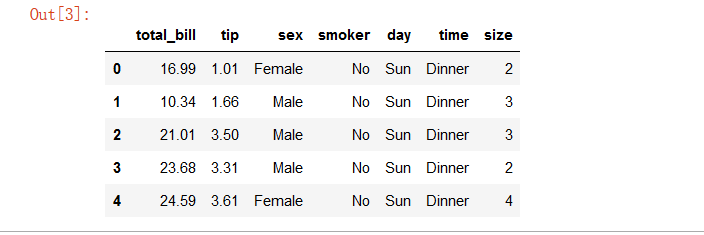### 分类散点图

sns.set(style='white', color_codes=True)
sns.stripplot(x='day', y='total_bill', data=tips)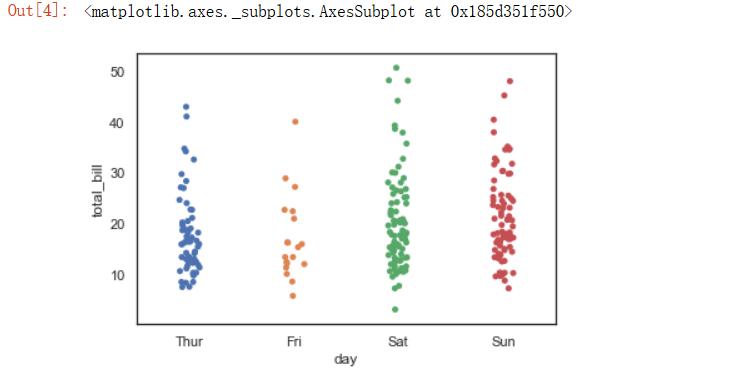sns.stripplot(x='day', y='total_bill', data=tips, jitter=True)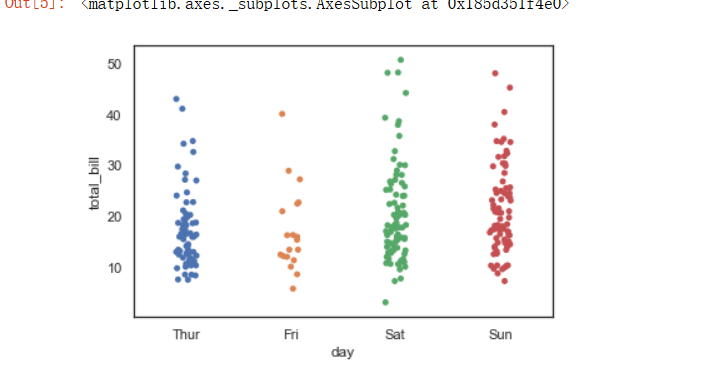sns.swarmplot(x='day', y='total_bill', data=tips)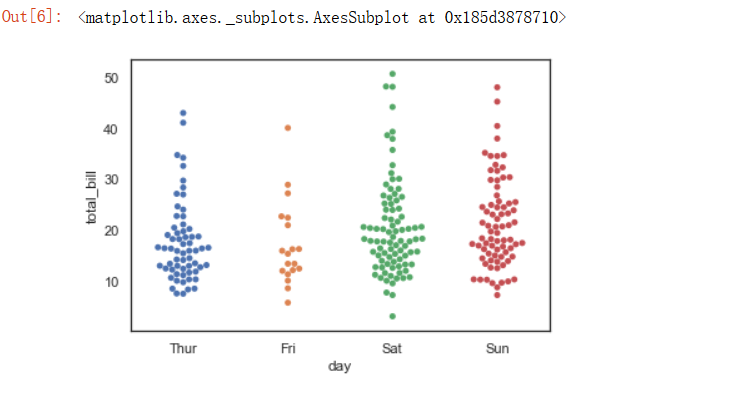通过swarmplot() 函数的hue 参数可以多嵌套一个分类变量，它会以不同的颜色在图表中呈现。

sns.swarmplot(x='day', y='total_bill', data=tips, hue='sex')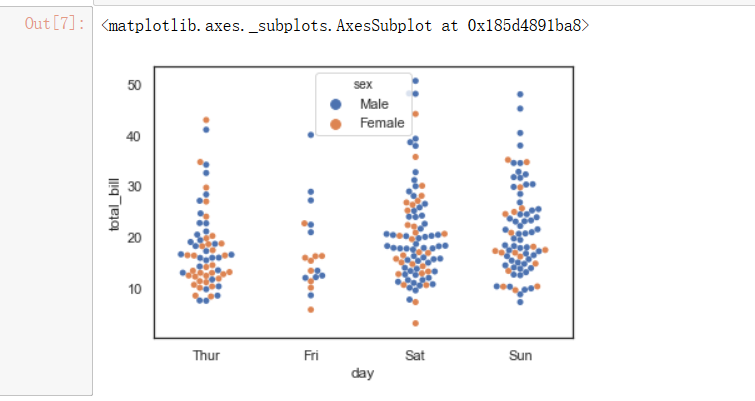09-116281
06-07240
12-264674
01-187万+
06-152412
06-15681
09-15
01-032万+
08-095万+